# An Optimal Quantitative PID Controller Design for Ball and Beam System

An Optimal Quantitative PID Controller Design for Ball and Beam System
Control and Systems Engineering Department, University of Technology-Iraq, Baghdad 10066, Iraq

Corresponding Author Email:
Page:
323-329
|
DOI:
https://doi.org/10.18280/jesa.550304
16 February 2022
|
Accepted:
25 April 2022
|
Published:
30 June 2022
| Citation

OPEN ACCESS

Abstract:

In this paper, the design of two degree of freedom controller for ball and beam system is proposed. The Quantitative Feedback Theory (QFT) constraints are used to design two PID controllers to stabilize and compensate the nonlinear system with robust stability, robust performance and more desirable time response specifications. A multi objective cost function is proposed in this work to represent the function of QFT. The Black Hole Optimization (BHO) technique is a useful method to automate the design procedure of the proposed controller. The results of simulation indicate that the suggested optimal quantitative PID controller can give a desirable performance in comparison to other controllers designed in previous work.

Keywords:

robust control, optimal control, PID controller, black hole optimization, ball and beam system

1. Introduction

Quantitative Feedback Theory (QFT) is an engineering methodology, which was developed by Horowitz (1960). It was designed to achieve the closed loop system requirements in the presence of system uncertainty. The fundamental idea is to convert model needs and plant uncertainty into open-loop, which are then used to construct a controller using the gain-phase loop shaping approach [1, 2].

On the other hand, PID controller is still the most significant controller, which is commonly used to solve many control problems. The ability to make the PID controller with automatic tuning, automatic development of gain schedules and continuous adaptation has renewed interest in PID control . One of the fixed structure controllers is a PID controller whose structure has been selected independently of the plant order [4-6].

Many control approaches have been used to control the system using a variety of design methodologies. Mehedi et al.  in 2018 proposed Fractional Order PD Control (FOC) to control the ball position. Ali et al.  in 2018 proposed PID controller to stabilize the system, PSO method was used to tune parameters of the controller. Shah et al.  in 2018 proposed LQR controller to control the system. Tahir et al.  in 2019 Proposed PID controller to control the system where the parameters tuned manual. Abdul Aziz et al.  in 2019 proposed a fuzzy PID controller for the system and the output reference is modified as required by applying a model reference at the input. The changes of system parameters did not taken into account. Tsoi et al.  in 2020 proposed a Genetic Algorithm (GA), the purpose of GA is to improve an extra layer of fuzziness. Many objective functions were analyzed to develop the performance of cost matrix ITAE, IAE and ISE. Two events bring the optimum parameter search to a close. The first was the achievement of the highest number of chromosomal generations, and the second stage was determining the optimal controller parameter solution. The result of ITAE cost function was better than the other cost functions. Amirudin and Kadir  in 2020, the location of the ball on the system was controlled using adaptive PID (Q-PID). The (Q-PID) was compared to traditional PID and heuristic PID controllers techniques. It was discovered that the adaptive PID which based on Learning, was better than conventional PID and heuristic PID controller techniques.

In this paper, optimal quantitative PID controller is proposed for the ball and beam system. The Black Hole Optimization (BHO) method is used to automate the procedure of (QFT). The method is used to automate the loop shaping and obtain the optimal PID controller subject to QFT constraints.

This paper is formatted as follows: The modeling of the system is presented in section 2. Section 3 explains the Black Hole Optimization (BHO) method. controller design is presented in section 4. In section Finally, the results and discussion are presented in section5 and the conclusion is given in section 6.

2. Ball and Beam Model

## 1.png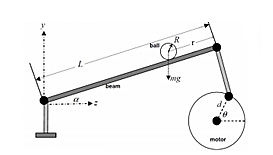Figure 1. Ball and beam system structure

The ball and beam is one of the mechanical systems which is commonly related to actual control issues, such as horizontally stabilizing the aircraft at the landing and turbulent airflow . The system consists of the ball, beam and the ends of the beam are attached with level arms The motor's output gear is attached to one of the level arms and the other level arm, which is fixed. The drawback of this configuration is that it necessitates a more excellent analysis of the mechanical components. It has high nonlinearity and uncertainty parameters [15, 16]. Figure 1. shows the system.

The following dynamic equation is created using the Newton method as :

$f_{t}+f_{r}=-m g \sin \alpha$            (1)

where, ft is the force produced by translational motion, fr is the force produced by rotational motion, g is the gravitational constant, and α is the angle of the beam.

Then, the force due to ball rotational, fr is found as: the torque produce by the rotational motion of the ball is equal to radius of the ball, R, multiplied by the rotational force as follows:

$T_{r}=F_{r} R$                  (2)

Also the torque can be written as its moment of inertia multiplied by the double derivative of its translational motion

$T_{r}=F_{r} R=\frac{J}{R} \ddot{r}$                (3)

where, J is moment of inertia, R is radius of the ball and $\ddot{r}$ is by the double derivative of its translational motion.

where, J is given as follow:

$J=\frac{2 m R^{2}}{5}$              (4)

The force due to ball rotational is given From Eq. (3) as follow:

$f_{r}=\left(\frac{\frac{2 m R^{2}}{5}}{R^{2}} \ddot{r}\right)$                  (5)

and

$f_{t}=m \ddot{r}$                 (6)

where, m represents the mass of the ball, R represent the radius of the ball and r is the ball position.

$\left(\frac{\frac{2 m R^{2}}{5}}{R^{2}}+m\right) \ddot{r}=-m g \sin \alpha$                  (7)

and

$\left(\frac{7}{5} m\right) \ddot{r}=-m g \sin \alpha$                  (8)

Then

$\ddot{r}=-\frac{5}{7} g \sin \alpha$                    (9)

The relationship between the beam angle (α) and the servo motor angle (θ) is shown in Figure 2. This relation can be represented using the geometry and trigonometry of the level arm section .

## 2.png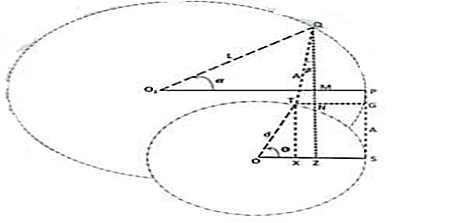Figure 2. The beam angle and the angle of servo motor 

By analyzing the vector (d), the following two components are obtained:

$O X=d \cos \theta ; T X=d \sin \theta$                   (10)

After subtracting OX from OS, the length of XS is obtained as:

$X S=d(1-\cos \theta)$                (11)

By analyzing the vector (L), the following two components are obtained:

$O_{1} M=L \cos \alpha ; Q M=L \sin \alpha$                  (12)

Then subtracting O1M from O1P, yields:

$M P=L(1-\cos \alpha)$                (13)

By analyzing the vector (A), the following two components are obtained:

$T N=A \sin \beta ; Q N=A \cos \beta$                  (14)

Then subtracting QM from QN, yields:

$M N=A \cos \beta-L \sin \alpha$                  (15)

$T X=M Z-M N$                  (16)

After substituting Eqns. (10) and (15) in Eq. (16), yields:

$L \sin \alpha=d \sin \theta-A(1-\cos \beta)$                  (17)

By multiplying both sides of Eq. (17) by arcsine, yields:

$\alpha=\arcsin \left[\frac{d}{L} \sin \theta-\frac{A}{L}(1-\cos \beta)\right]$                  (18)

$T N+N G=X S$                (19)

By multiplying both sides of Eq. (19) by arcsine, yields:

$\alpha=\arcsin \left[\frac{d}{L} \sin \theta\right]$                   (20)

Then, the relationship between the beam angle (α) and the servo motor angle (θ) can be expressed by:

$\alpha=\frac{d}{L} \theta$                 (21)

where, d is the level arm, L represent the beam length, α(s) is the beam angle, and θ(s) is the gear angle.

Substituting Eq. (21) in Eq. (9), yields:

$\ddot{r}=G_{b b}=-\frac{5}{7} g \sin \frac{d}{L} \theta$                  (22)

The electric equation for the motor is given as :

$\ddot{\theta}=\frac{k_{m} k_{g}}{R_{a} J e q}\left(v_{a}-k_{b} k_{g} \dot{\theta}\right)$                 (23)

where, km represent a motor torque, kg represent a gear ratio, kb represent a back emf constant, Ra represent motor resistance and Jeq is the equivalent inertia.

From Eq. (9), the relationship between the motor angle and voltage of the motor can be obtained as:

$\frac{\theta(s)}{v_{a}(s)}=\frac{\frac{k_{m} k_{g}}{R_{a} {J e q}}}{s^{2}+\frac{K_{m} K_{g} K_{b} {K}_g}{R_{a J e q}} s}$                  (24)

The parameters of the ball and beam system are given in Table 1.

Table 1. Parameters of ball and beam system 

 Parameters Value Gear ratio 70:1 Motor torque constant 0.0076 Nm/A Beam length 43.18 cm Level radius 2.54 cm Equivalent inertia 0.0029 kg.m2 Motor resistance 2.6 Ω Mass of ball 0.11 kg Constant of Gravitational 9.8 m/s2 Constant of Back emf 0.00767 v/(rad/sec)
3. Black Hole Optimization (BHO) Method

Black Hole optimization is the population based approach to some mechanisms to solve the problem . To implement Black Hole, initial population must be generated, and then the value of fitness is calculated for each candidate. If the candidate has a best fitness namely Black Hole, and other namely stars, stars that surround Black Hole can be absorbed by Black Hole .

The following is the formula for star absorption by a Black Hole :

$x_{s}(t+1)=x_{s}(t)+\operatorname{rand} \times\left(x_{b}(t)-x_{s}(t)\right)$                   (25)

where, xs represent location of star solution and xb represents the black hole.

When the stars move toward a black hole, they may also exceed event horizon. Each star that passes over event horizon of black hole will be pulled in . A candidate (star) being pulled into the Black Hole, A new candidate solution (star) is created and scattered at random throughout search area, and the new search is initiated. After all of the stars have been relocated, the next iteration begins. In the Black Hole algorithm, the radius of the event horizon is determined using :

$C=\frac{z}{\sum_{i}^{n} z_{C}}$                     (26)

where, z represents the value of fitness for Black Hole, zC represent the value of fitness for candidates and n represent the number of candidates solutions. Figure 3 shows the flowchart of BHO.

## 3.png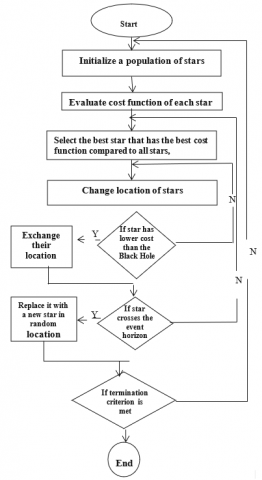Figure 3. The flowchart of BHO 

4. Controller Design

In this section the procedure to design the proposed optimal quantitative PID controller is given.

4.1 Quantitative PID controller design

The proposed controller parameters are tuned by BHO subject to Quantitative Feedback Theory (QFT). The suggested objective is to satisfy the following QFT requirements:

1. To satisfy the performance requirements by making the closed-loop response fall between the given upper tracking and lower tracking performances. Upper tracking and lower tracking bounds are known structure transfer functions with parameters adjusted to meet the requirements. The suggested lower tracking bound and upper tracking bound are:

$T_{u}=\frac{(4 s+4)}{\left(s^{2}+4 s+4\right)}$                  (27)

$T_{1}=\frac{4}{(s+2)(s+2)(1+0.2 s)}$                     (28)

The specifications of the proposed upper tracking are: rise time equals to 0.3 sec, settling time equals to 2 sec, overshoot equals 0.135 and the specifications of the proposed lower tracking are: rise time equals to 1.7 sec, settling time equals to 3 sec and overshoot equals zero.

To satisfy this constraint the following variation must be minimize:

$\delta_{n}\left(j w_{i}\right)=\left|\frac{T_{u}\left(j w_{i}\right)-T_{L}\left(j w_{i}\right)}{2}\right|-\left|\frac{F\left(j w_{i}\right) K\left(j w_{i}\right) G_{p}\left(j w_{i}\right)}{1+K\left(j w_{i}\right) G_{p}\left(j w_{i}\right)}\right|$                   (29)

2. To achieve the robust stability for the controlled system by considering [24, 25]:

$\left|\frac{K(j w) G(j w)}{1+K(j w) G(j w)}\right| \leq M_{r}$                      (30)

where, K(jw) represent the controller and Mr represent a constant which is obtained according to the desired gain margin and phase margin. They are given as:

$G M=1+\frac{1}{M_{r}}$                   (31)

$\mathrm{PM}=\cos ^{-1}\left(1-\frac{1}{2 M_{r}^{2}}\right)$                   (32)

3. To obtain $\delta_{\mathrm{cl}}\left(j \mathrm{w}_{\mathrm{i}}\right)$ for the controlled system which being smaller than or equal to $\delta_{\mathrm{v}}\left(\mathrm{jw}_{\mathrm{i}}\right)$ that it equals or less than $\delta_{\mathrm{d}}\left(\mathrm{jw}_{\mathrm{i}}\right)$ for each value of wi as:

$\delta_{c l}\left(j w_{i}\right) \leq \delta_{v}\left(j w_{i}\right) \leq \delta_{d}\left(j w_{i}\right)$                    (33)

where, δcl(jwi) represents maximum variation in magnitude for closed loop controlled system with uncertainty, δv(jwi) represents difference between magnitudes of the upper tracking and lower tracking boundaries and δd(jwi) represents the maximum variation in magnitudes due to the system parameters uncertainty. These relations can be expressed as:

$\delta_{\mathrm{d}}\left(j w_{\mathrm{i}}\right)=\left|\widehat{\mathrm{G}}\left(j w_{\mathrm{i}}\right)\right|-\left|\mathrm{G}\left(j w_{\mathrm{i}}\right)\right|$                 (34)

$\delta_{\mathrm{v}}\left(j w_{\mathrm{i}}\right)=\left|\mathrm{T}_{\mathrm{u}}\left(j w_{\mathrm{i}}\right)\right|-\left|\mathrm{T}_{\mathrm{l}}\left(j w_{\mathrm{i}}\right)\right|$                     (35)

where, $\widehat{\mathrm{G}}(j w_{\mathrm{i}})$ represents the overall uncertain system.

4. To achieve good disturbance rejection by minimizing the sensitivity function.

From all previously mentioned QFT constraints, the following cost function can be proposed:

$J(h)=\left|\delta_{c l}\left(j w_{i}\right)\right|+\left|\delta_{n}\left(j w_{i}\right)\right|+\left|S\left(j w_{i}\right)\right|+\int_{0}^{t_{f}} e^{2}(t) d t$                (36)

4.2 Tuning of PID controllers

In this paper, two degree of freedom controllers shown in Figure 4 are used to control the ball and beam system. Inner loop controller is to control a motor angle. The inner controller must be designed in such a way that motor angle follows the reference signal. As shown in Figure 5, the outer loop uses the inner loop to control a ball position. The structures of both controllers are:

$K_{1}(s)=k_{p 1}+\frac{k_{i 1}}{s}+k_{d 1} s$                    (37)

$K_{2}(s)=k_{p 2}+\frac{k_{i 2}}{s}+k_{d 2} s$                    (38)

where, K1(s) and K2(s) represent the inner loop and outer loop controllers.

And

$F(s)=\frac{1}{\left(\tau_{1} s+1\right)\left(\tau_{2} s+1\right)}$                    (39)

where, kp1, ki1, kd1, kp2, ki2, kd2, represent the controllers' parameters and τ1, τ2 are the time constants of the prefilter which are tuned by BHO method subject to QFT constraints as shown in Figure 6.

## 4.png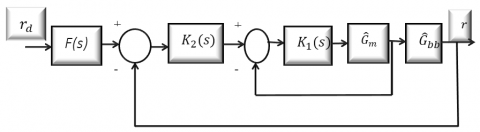Figure 4. Block diagram of the controlled ball and beam system

where, K1(s) and K2(s) represent two controllers to be designed, F(s) is the prefilter, rd is desired position and r is actual position.

## 5.png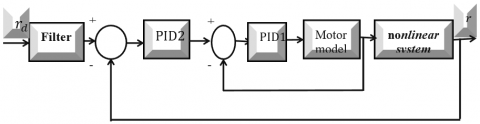Figure 5. Block diagram of Ball and Beam system

## 6.png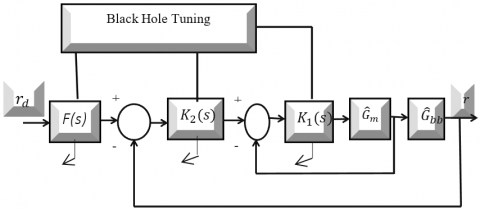Figure 6. Block diagram of ball and beam system with Black Hole

The setting of the Black Hole Optimization method to obtain the optimal parameters of the controllers is given as:

1. The number of dimensions is 8.

2. Initial population is 50.

3. Maximum iteration is 50.

After tuning the controller parameters by Black Hole Optimization subject to Quantitative Feedback (QFT), the controllers and prefilter are obtained as:

$k_{1}(s)=7+\frac{0.003}{s}+0.017 s$                   (40)

$k_{2}(s)=0.003+\frac{0.003}{s}+5 s$                    (41)

$F(s)=\frac{1}{(0.24 s+1)(0.03 s+1)}$                  (42)

5. Results and Discussion

The desired performance requirements have been satisfied by applying proposed controller. An achieved time response specifications are: rise time equals 0.9 sec, settling time equals 1.8 sec, and overshoot is zero, as shown in Figure 7. This means that the constraints of QFT represented by Eq. (36) have been achieved. It is clear that the resulting steady state error is zero which is the main advantage of the integral term in PID controller. The nominal system lies between the desired upper tracking and lower tracking boundaries. Figure 8 shows the time response of the system.

## 7.pngFigure 7. The ball position

## 8.png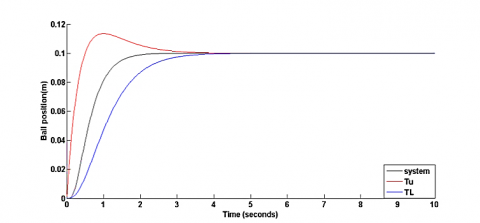Figure 8. Time response of the system

## 9.png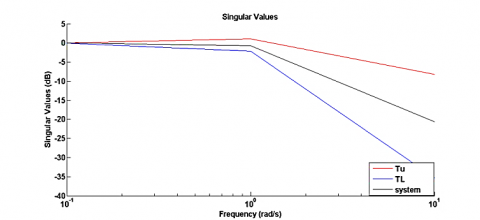Figure 9. Ball position in the frequency domain

The constraints for robust stability have been achieved where the nominal system lies between the upper tracking and lower tracking boundaries Figure 9 shows ball position in frequency domain. It is obvious that the response of the nonlinear system with uncertain parameters lies between the given tracking boundaries as shown in Figure 10. Also, it was found that the response of the Figure 11 shows the response of the uncertain system in the frequency domain. It is clear that the robustness requirements were satisfied by the proposed controllers.

The obtained control signal is shown in Figure 12 it is shown that a low control voltage is required to control the ball and beam system. Table 2 contains a comparison between the Optimal Quantitative PID Controller and fuzzy PID controller.

It is shown that the suggested optimal quantitative PID controller can achieve a desirable performance.

## 10.png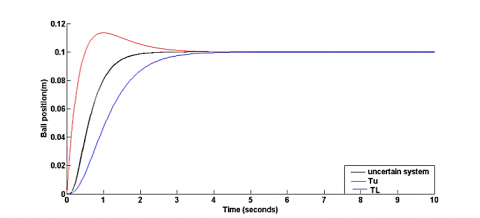Figure 10. Time response of the uncertain system

## 11.png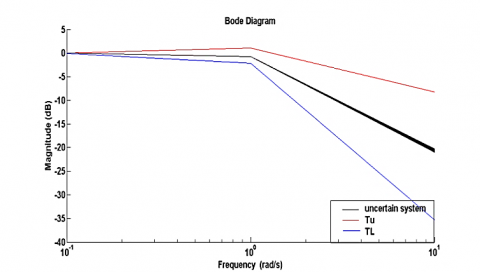Figure 11. Frequency response properties of uncertain system

## 12.pngFigure 12. Control signal

Table 2. Comparison between the optimal quantitative PID controller and fuzzy PID controller

 Controller Settling time (sec) Rise Time (sec) Overshoot (%) Fuzzy PID  11.95 6.97 0 An Optimal Quantitative PID 1.8 0.9 0
6. Conclusions

In this work, the Black Hole Optimization method was utilized to develop a quantitative PID controller for the ball and beam system. The controller parameters were obtained subject to QFT constraints. The results showed that the QFT constraints for robust stability and performance have been achieved. Moreover, it was found that the proposed quantitative PID controller could compensate effectively the nonlinear system with parameters uncertainty. It was shown that the quantitative PID has satisfied the more desirable performance in comparison to a fuzzy PID controller.

Nomenclature
 d Level arm, cm g gravitational acceleration, m.s-2 Jeq the equivalent inertia, kg.m2 km a motor torque Nm/A kg Gear ratio kb Back emf constant, v/(rad/sec) L the beam length, cm m mass of the ball kg r the ball position, m Ra motor resistance, $\Omega$ Greek symbols $\alpha(s)$ Beam angle $\theta(s)$ Gear angle Subscripts BHO Black Hole Optimization PID Proportional- Integral-Derivative QFT Quantitative Feedback Theory
References

 Ali, H.I., Noor, S.B.B.M., Bashi, S.M., Marhaban, M.H. (2012). Quantitative feedback theory control design using particle swarm optimization method. Transactions of the Institute of Measurement and Control, 34(4): 463-476. https://doi.org/10.1177/0142331210397084

 Kobaku, T., Jeyasenthil, R., Sahoo, S., Ramchand, R., Dragicevic, T. (2020). Quantitative feedback design-based robust PID control of voltage mode controlled DC-DC boost converter. IEEE Transactions on Circuits and Systems II: Express Briefs, 68(1): 286-290. https://doi.org/10.1109/TCSII.2020.2988319

 Ali, H.I., Noor, S.M., Bashi, S.M., Marhaban, M.H. (2010). Design of H-infinity based robust control algorithms using particle swarm optimization method. Mediterr. J. Measur. Control, 6(2): 70-81.

 Ali, H.I. (2014). Robust PI-PD controller design for magnetic levitation system. Engineering and Technology Journal, 32(3): 667-680.

 Ali, H.I., Saeed, A.H. (2016). Robust PI-PD controller design for systems with parametric uncertainties. Engineering & Technology Journal, 34: 2167-2173.

 Ali, H.I., Abd, M.I. (2015). Optimal multi-objective robust controller design for magnetic levitation system. Iraqi Journals of Computers, Communications, Control and Systems Engineering, 15(1): 13-34.

 Mehedi, I.M., Al-Saggaf, U.M., Mansouri, R., Bettayeb, M. (2019). Two degrees of freedom fractional controller design: Application to the ball and beam system. Measurement, 135: 13-22. https://doi.org/10.1016/j.measurement.2018.11.021

 Ali, H., Albagul, A., Algitta, A. (2020). Optimization of PID parameters based on Particle Swarm optimization for ball and beam system. Int. J. Eng. Technol. Manag. Res, 5(9): 59-69. https://doi.org/10.5281/zenodo.1443549

 Shah, M., Ali, R., Malik, F.M. (2018). Control of ball and beam with LQR control scheme using flatness based approach. In 2018 International Conference on Computing, Electronic and Electrical Engineering (ICE Cube), pp. 1-5. https://doi.org/10.1109/ICECUBE.2018.8610968

 Tahir, N.M., Yekinni, L.A., Bature, U.I., Yahuza, I., Shuaibu, A.N., Sambo, A.U. (2019). Control and Stability Studies of Ball and Beam System. In Proc. of the Journal of Modern Technology and Engineering, 4(2): 122-131.

 Aziz, N.S.A., Rahiman, M.H.F., Ishak, N., Adnan, R., Tajjudin, M. (2019). Design of fuzzy PID controller with reference model for ball and beam mechanism. In 2019 IEEE International Conference on Automatic Control and Intelligent Systems (I2CACIS), pp. 194-198. https://doi.org/10.1109/I2CACIS.2019.8825012

 Tsoi, J.K., Patel, N.D., Swain, A.K., Huang, X. (2020). Dynamic fuzzy membership intervals with two-stage objective function for ball and beam system based on GA tuning. In 2020 16th International Conference on Control, Automation, Robotics and Vision (ICARCV), pp. 898-903. https://doi.org/10.1109/ICARCV50220.2020.9305475

 Amiruddin, B.P., Kadir, R.E.A. (2020). Ball and Beam Control using adaptive PID based on Q-Learning. In 2020 7th International Conference on Electrical Engineering, Computer Sciences and Informatics (EECSI), pp. 203-208. https://doi.org/10.23919/EECSI50503.2020.9251898

 Ali, A.T., Ahmed, A.M., Almahdi, H.A., Taha, O.A., Naseraldeen, A. (2017). Design and implementation of ball and beam system using PID controller. MAYFEB Journal of Electrical and Computer Engineering, 3(1): 1-4.

 Shirke, H.R., Kulkarni, N.R. (2015). Mathematical modeling, simulation and control of ball and beam system. International Journal of Engineering Research & Technology, 4(3): 834-838.

 Soni, R. (2018). Optimal control of a ball and beam system through LQR and LQG. In 2018 2nd International Conference on Inventive Systems and Control (ICISC), pp. 179-184. https://doi.org/10.1109/ICISC.2018.8399060

 Sharma, J., Pratap, B. (2015). Quantitative feedback theory based control of ball and beam system with parametric uncertainty. In 2015 IEEE Students Conference on Engineering and Systems (SCES), pp. 1-6. https://doi.org/10.1109/SCES.2015.7506443

 Anand. S., Prasad. R. (2017). Modeling and control of Ball and Beam system. International Journal of Engineering Research in Electronics and Communication Engineering (IJERECE), 4(9): 1-7.

 Abdulwahab, H.A., Noraziah, A., Alsewari, A.A., Salih, S.Q. (2019). An enhanced version of black hole algorithm via levy flight for optimization and data clustering problems. IEEE Access, 7: 142085-142096. https://doi.org/10.1109/ACCESS.2019.2937021

 Soto, R., Crawford, B., Olivares, R., Taramasco, C., Figueroa, I., Gómez, Á., Paredes, F. (2018). Adaptive black hole algorithm for solving the set covering problem. Mathematical Problems in Engineering, 2018: 2183214. https://doi.org/10.1155/2018/2183214

 Hatamlou, A. (2013). Black hole: A new heuristic optimization approach for data clustering. Information Sciences, 222: 175-184. https://doi.org/10.1016/j.ins.2012.08.023

 Arenas-Acuña, C.A., Rodriguez-Contreras, J.A., Montoya, O.D., Rivas-Trujillo, E. (2021). Black-Hole optimization applied to the parametric estimation in distribution transformers considering voltage and current measures. Computers, 10(10): 124. https://doi.org/10.3390/computers10100124

 Ali, H.I., Hadi, M.A. (2020). Optimal nonlinear controller design for different classes of nonlinear systems using black hole optimization method. Arabian Journal for Science and Engineering, 45(8): 7033-7053. https://doi.org/10.1007/s13369-020-04650-z

 Ali, H.I. (2012). Hybrid H-infinity/QFT robust control algorithm design using PSO for positioning a pneumatic servo actuator system. The Mediterranean Journal of Measurement and Control, 8(2): 413-430.

 Moghadam, A.A.A., Marshall, M. (2021). Robust control of the flywheel inverted pendulum system considering parameter uncertainty. In 2021 American Control Conference (ACC), pp. 1730-1735. https://doi.org/10.23919/ACC50511.2021.9483178